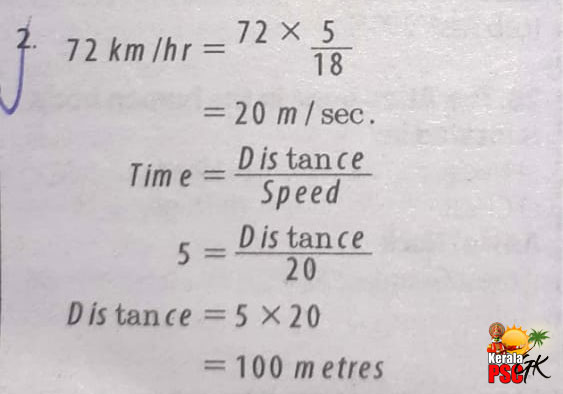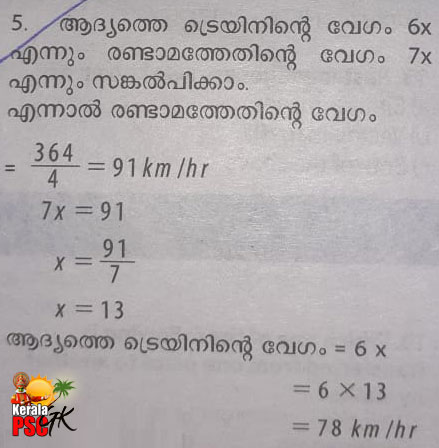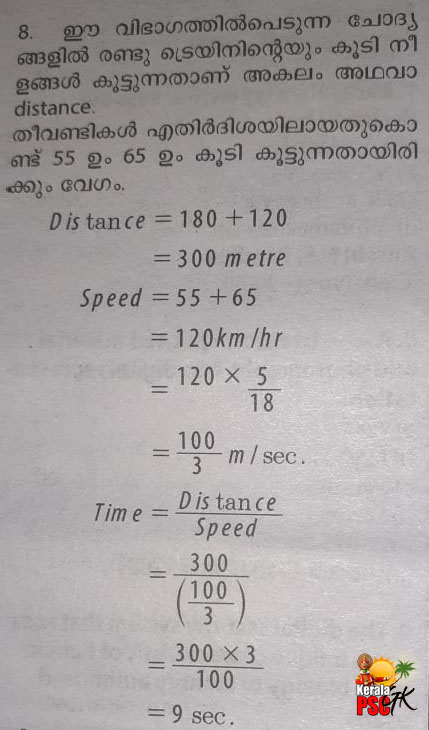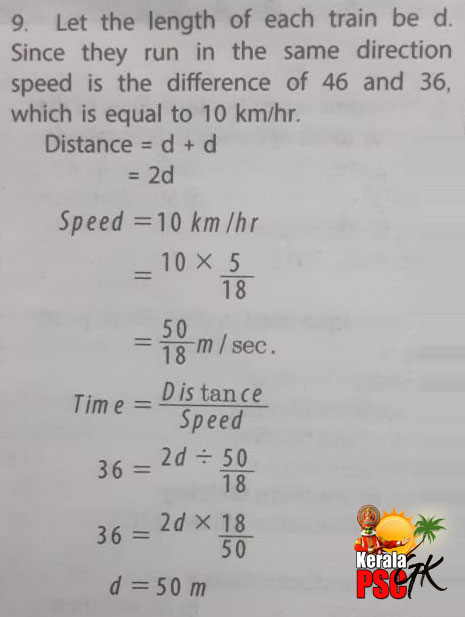# Kerala PSC GK | Practice/Model Maths Questions - 3

1. A train is travelling at the rate of 45 km/hr. The time taken by the Train to cover a distance of ⅘ km is:
[a] 36 Seconds
[b] 64 Seconds
[c] 90 Seconds
[d] 120 Seconds2. The speed of a bus is 72 km/hr. The distance covered by the bus in 5 seconds is:
[a] 100 m
[b] 60 m
[c] 50 m
[d] 74.5 m3. A man goes from A to B at a uniform speed of 12 km/hr and returns with a uniform speed of 4 km/hr, his average speed for the whole journey is:
[a] 8 km/hr
[b] 7.5 km/hr
[c] 6 km/hr
[d] 4.5 km/hr4. A man goes to a place on bicycle at a speed of 16 km/hr and comes back at lower speed. If the average speed is 6.4 km/hr in total journey, then the return speed is
[a] 10 km/hr
[b] 8 km/hr
[c] 6 km/hr
[d] 4 km/hr5. The speeds of two trains are in the ratio 6:7. If the second trains runs 364 km in 4 hours, then the speed of the first train is:
[a] 60 km/hr
[b] 72 km/hr
[c] 78 km/hr
[d] 84 km/hr6. A train covers the distance between station A and B in 45 minutes. If the speed of the train is reduced by 5 km/hr, then the same distance is covered in 48minutes. The distance between station A and B is:
[a] 60 km
[b] 64 km
[c] 80 km
[d] 55 km7. Two trains start from a certain place on two parallel tracks in the same direction. The speed of the trains are 45 km/hr and 40 km/hr respectively. The distance between the trains after 45 minutes will be
[a] 2 km 500 m
[b] 2 km 750 m
[c] 3 km 750 m
[d] 3 km 250 m8. Two trains 180 metres and 120 metres in length are running towards each other on parallel tracks one at the rate 65 km/hr and another at 55 km/hr. In how many seconds will they be clear of each other from the moment they meet?
[a] 6
[b] 9
[c] 12
[d] 159. Two trains of equal length are running on parallel lines in the same direction at 46 km/hr and 36 km/hr. The faster train passes the slower train in 36 seconds. The length of each train is:
[a] 72 m
[b] 80 m
[c] 82 m
[d] 50 m10. A train is moving at a speed of 132 km/hr. If the length of the train is 110 metres, how long willit take to cross a railway platform 165 metres long?
[a] 5 sec
[b] 7.5 sec
[c] 10 sec
[d] 15 sec